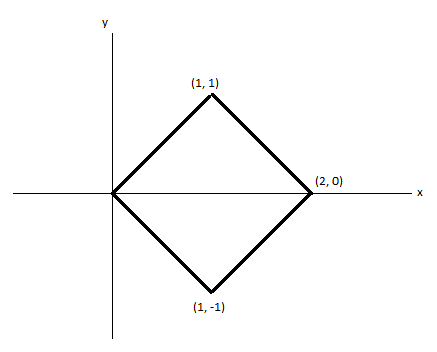# Use the transformation \begin{array}{l}{x=u+v} \\ {y=u-v}\end{array} to evaluate ...

## Question:

Use the transformation

{eq}\begin{array}{l}{x=u+v} \\ {y=u-v}\end{array} {/eq}

to evaluate {eq}\iint_{\mathbb{R}}(x+2 y) d A_{x, y} {/eq} where R is the region in the xy-plane shown below.Note: You may use the fact that the inverse transformation is

{eq}\begin{array}{l}{u=\frac{x+y}{2}} \\ {v=\frac{x-y}{2}}\end{array} {/eq}

## Double Integral:

We will solve the problem by changing it to u and v form here the region will become rectangle and then we will integrate it along the rectangle.

To solve the problem we will use the variable separable form:

{eq}\int \int (x+2y)dxdy {/eq}

Now let us substitute:

{eq}x=u+v\\ y=u-v\\ u=\frac{x+y}{2}\\ v=\frac{x-y}{2} {/eq}

Now let us find the Jacobian:

{eq}\begin{bmatrix} \frac{\partial x}{\partial u} & \frac{\partial x}{\partial v}\\ \frac{\partial y}{\partial u} & \frac{\partial y}{\partial v} \end{bmatrix}\\ =\begin{bmatrix} 1 &1 \\ 1 & -1 \end{bmatrix}\\ =-2 {/eq}

The integral will be:

{eq}=-2 {/eq}